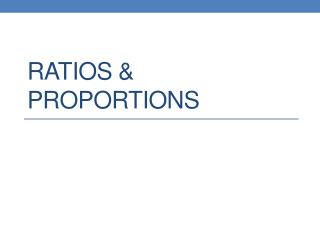Download PresentationRatios & proportions

Ratios & proportions - PowerPoint PPT Presentation

Ratios & proportions. Ratio. A comparison of two numbers by division. The ratio of x to y can be written: X to y X : Y. What is the ratio of boys to puppies?. Proportions. An equation stating that two ratios are equal. Write a proportion….I am the owner, or an agent authorized to act on behalf of the owner, of the copyrighted work described.
Download PresentationRatios & proportions

Download Policy: Content on the Website is provided to you AS IS for your information and personal use and may not be sold / licensed / shared on other websites without getting consent from its author.While downloading, if for some reason you are not able to download a presentation, the publisher may have deleted the file from their server.

- - - - - - - - - - - - - - - - - - - - - - - - - - E N D - - - - - - - - - - - - - - - - - - - - - - - - - -
Presentation Transcript
1. Ratios & proportions

2. Ratio • A comparison of two numbers by division. • The ratio of x to y can be written: • X to y • X : Y

3. What is the ratio of boys to puppies?

4. Proportions • An equation stating that two ratios are equal.

5. Write a proportion….

6. Simplify the Following Ratios • 9:15 • 60: 84

7. Simplify the Following Ratios • .25 : .8

8. Simplify the Following Ratios

9. Six students in a class of 32 are absent. Find the ratio of… • The number absent to the number present. • The number absent to the total number.

10. Two baby snakes are measured in the zoo. One measures 8cm and the other measures 12cm. Two weeks later they are measured again and the first one now measures 11cm while the second one measures 15cm. Did the snakes grow in proportion? Explain your answer.

11. Product of the Means = Product of the Extremes If and then

12. Use Cross Products to determine whether each pair of ratios forms a proportion.

13. Use Cross Products to determine whether each pair of ratios forms a proportion.

14. Solve:

15. Solve:

16. Solve:

17. Solve:

18. Solve:

19. Solve:

20. Luke bought 10 pens for €2.40. What would 12 pens cost him at the same rate? Show two methods of working out the answer.

21. Homework • Page 115 (9-42)